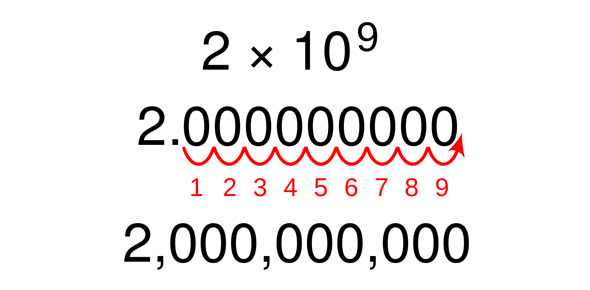# How To Use Numbers In Scientific Notation

6 Questions | Total Attempts: 62SettingsDo you know how to use numbers in scientific notation? Let's check now by taking this quiz!

• 1.
1. change to scientific: 6.800,000 =
• A.

6.800* 10^7

• B.

6.800*10^6

• C.

68.000*10^6

• 2.
2. 1.46800 = 1.468*108
• A.

True

• B.

False

• 3.
3. change to standard: 6.0 * 103
• A.

6.0000

• B.

6.8000

• C.

6.000

• 4.
4. 6.543*105  = 6.54300
• A.

True

• B.

False

• 5.
5. Is a way to write very long or very small numbers.
• 6.
6. change to scientific:  7.896,000,000
• A.

7.896*10^9

• B.

7.896*10^7

• C.

7.896*10^5

Related TopicsBack to top